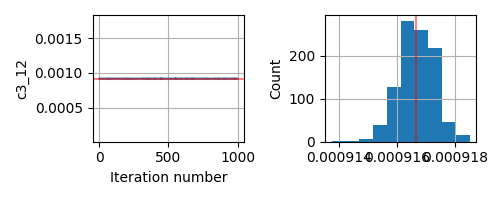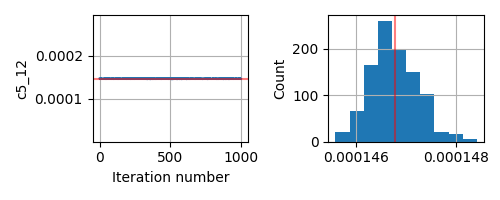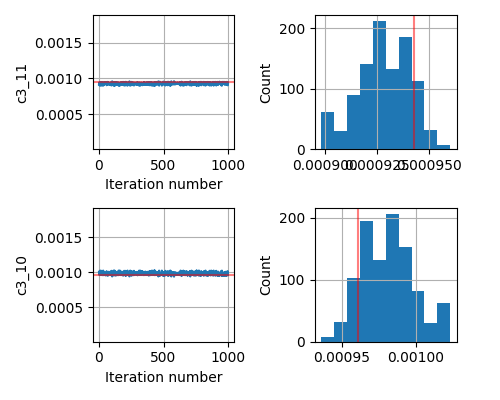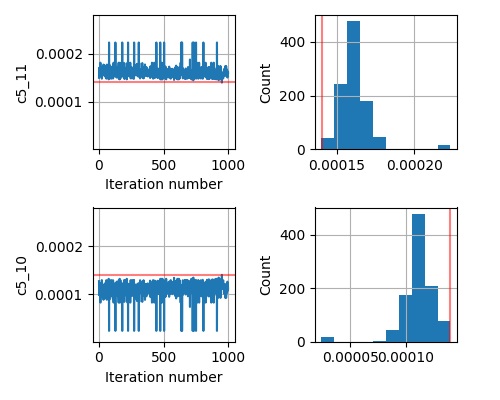# SA not converging to correct answer

I am working on a possibly high dimensional problem (somewhere between 45-60 parameters). Since the forward problem is expensive (involves eigenvalue problem) we are using relatively faster kernels like SA instead of NUTS.

1. We first set up a synthetic data from the forward problem that we have, so that there is no noise and we can test if the SA kernel works perfectly. This did not work when we threw the entire set of parameters at the code.
2. Then we reduced the number of parameters to 2 (holding all the others fixed to their “true” value as used in generating the synthetic data). Doing this, the default SA failed to converge again.
3. Next we changed the `adapt_state_size` to 100 in the `SA` kernel. This successfully returns the 2 parameters from the simulation.
4. We then started slowly increasing the number of parameters to 4. But this failed again. Even with `adapt_state_size = 100`.

The current model we are testing with is exceptionally simple (does not involve an eigenvalue problem):

``````def model():
# sampling from a uniform prior
c0 = numpyro.sample(f'c0', dist.Uniform(cmin, cmax))
c1 = numpyro.sample(f'c1', dist.Uniform(cmin, cmax))
c2 = numpyro.sample(f'c2', dist.Uniform(cmin, cmax))
c3 = numpyro.sample(f'c3', dist.Uniform(cmin, cmax))

# the forward problem
pred = fixed_part + param_coeff * c0\
+ param_coeff * c1\
+ param_coeff * c2\
+ param_coeff * c3

pred = pred - data

return numpyro.factor('obs',
dist.Normal(pred, jnp.ones_like(pred)).\
log_prob(jnp.zeros_like(data)))
``````

As an added info, the terrain of our minimizing function (or likelihood function) for different values of any such parameter `c` looks like a very sharp gaussian.

This seems to be a relatively simple problem that `SA` should be able to handle. But even if I change the prior from Uniform to Normal centered around the true parameter, the inversion does not give me the correct answer.

What am I doing wrong? Should I rescale my parameter values? They are of the magnitudes of the order 1e-2 and 1e-3. Should I change the kernel? Should I add some other key in the kernel or the MCMC function call?

i generally wouldn’t expect SA to work well in high dimensional problems. it probably works best in fewer than 10-20 dimensions. note also that it requires lots of warm-up samples and lots of post-warmup samples, i.e. in the hundreds of thousands.

you might play around with SA and gaussian targets to get some intuition on what regimes it’s expected to work in and how many samples you’re likely to need.

Thanks @martinjankowiak for the answer. So, what is my best bet in terms of kernels which works well in such high dimensional parameter space as well as is fast?

unfortunately i don’t have a magic wand. generally gradient-based methods like NUTS are your best generic bet.

Providing some plots demonstrating what we observe. We have two kinds of model params: `c3_i` and `c5_i`. In this demonstration, we use `i=10, 11, 12`.

1. The minimizing function terrain looks like the following (left is the log likelihood and right is the likelihood). As you see it is a very nicely peaked gaussian. All the model params have similar terrains (the grid to obtain this varies from 0.0 * true_param to 2.0 * true_param, so 1.0 shows the location of the true model parameter).

2. For a `i=12` parameter inversion, it looks great. Left: The chain and Right: The histogram. The red line shows the true parameter. It looks the same for `i=10, 11` too. So, nice results so far!3. For `i=10,11`. We can see it already starts going bad. Note, these cases worked great in the `i=12` case (or standalone `I=10` or standalone `i=11`)
=4. For `i=10,11,12`. This is quite bad.

We have used `num_warmup=5500` and `num_samples=1000` for all the above. `adapt_state_size=100` and a uniform prior (which is much larger than the x-lim of the plots).

This example shows a maximum of 6 parameter inversion. I guess SA can handle that with such a nicely peaked gaussian likelihood? What are we doing wrong?

like i said above SA lots and lots of sample to adapt to the distribution and then explore it. 6500 samples is far far too few for this algorithm